# 如何在 Microsoft Excel 中使用 SUMIF

525次阅读## 将 SUMIF 用于单个单元格范围

``=SUMIF(C2:C7,">25000")``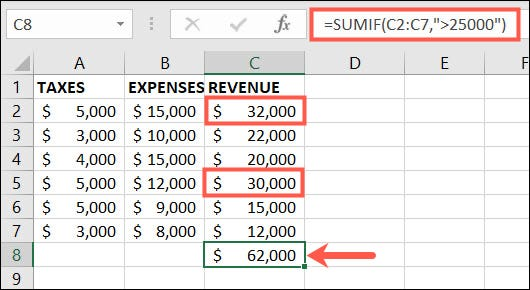``=SUMIF(B2:B7,"<10000")``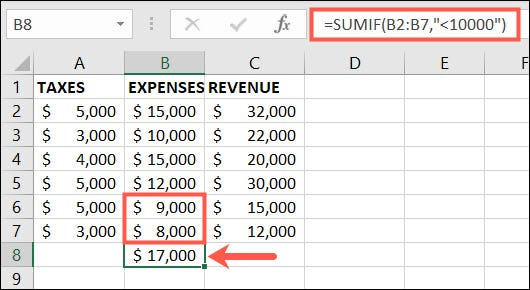``=SUMIF(A2:A7,"5000")``## 将 SUMIF 与数字标准一起用于多个范围

``=SUMIF(B2:B7,"<10000",C2:C7)``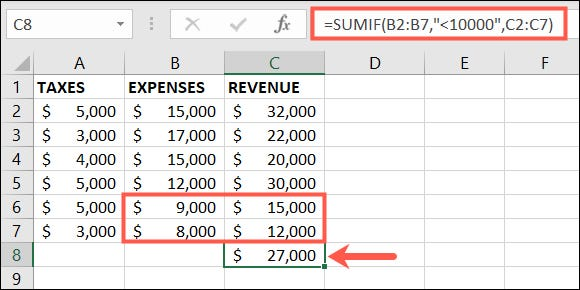``=SUMIF(C2:C7,">25000",B2:B7)``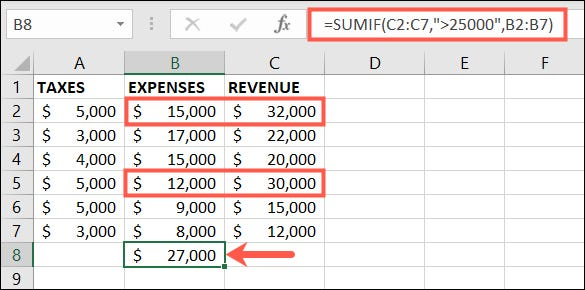``=SUMIF(C2:C7,">"&D2,B2:B7)``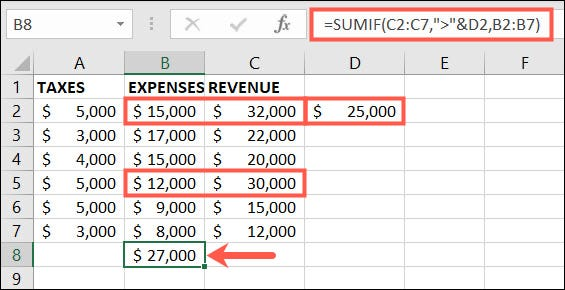## 将 SUMIF 与多个范围的文本条件结合使用

``=SUMIF(A2:B7,"服装",C2:C7)``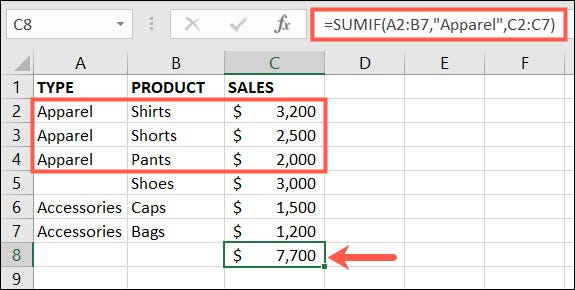``=SUMIF(B2:B7,"*ts",C2:C7)``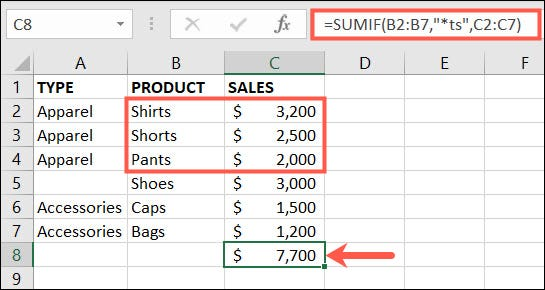``=SUMIF(A2:B7,"",C2:C7)``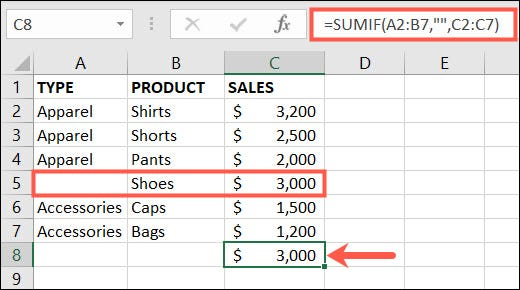Excel 中的 SUMIF 函数允许您采用基本方程并对其进行调整以满足您的需要。当添加数字不像二加二那么简单时，它非常方便。